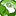# New to Qlik Sense

If you’re new to Qlik Sense, start with this Discussion Board and get up-to-speed quickly.

Announcements
Qlik Insider: SaaS capabilities for Data Integration and Analytics, May 25th! Register Now
cancel
Showing results for
Did you mean:Contributor II

## Exclude rows from a straight table

Hi,

maybe this is an easy task for someone, but I cannot solve it. In short, I have a straight table in QS and I want to exclude the rows if:

- the user is listed twice and has 'Yes' in AccessDenied for both systems (L and S),

- the user is listed once and has 'Yes'  in AccessDenied for the system used (L or S).

Users can be listed once or twice on the basis of the the system they use. In the screenshot attached, you can see a simplified version of my table, anyway the only user excluded would be User1 because it has 'Yes' for both system.

I tried to use the Previous function after sorting the rows by Full Name, now I'm trying something else at script level. Any idea on how I can do this? Thanks1 Solution

Accepted SolutionsMVP

There are two options

1) Create a dummy expression just so that you can hide some rows

``Avg({<[Full Name] = e({<[Full Name = {"=Concat(DISTINCT AccessDenied) = 'Yes'"}>})>} 1)``

2) Use calculated dimension which would replace your Full Name dimension

``````Aggr(
If(Concat(DISTINCT AccessDenied) <> 'Yes', [Full Name])
, [Full Name])``````
7 RepliesMVP

May be using  a set analysis like this

``{<[Full Name] = e({<[Full Name = {"=Concat(DISTINCT AccessDenied) = 'Yes'"}>})>}``Contributor II
Author

Thanks Sunny. I tried it, but it doesn't work. I see this error Garbage after expression: '='

Maybe the issue is related to the single and double quotes close to each other?MVP

I hope you are not using the above expression as is... This needs to part of an expression... for example... if you used Sum(Measure), then change it to

``Sum({<[Full Name] = e({<[Full Name = {"=Concat(DISTINCT AccessDenied) = 'Yes'"}>})>} Measure)``Contributor II
Author

In my table there are no measures, just several other flag fields and dates. That's the point.MVP

There are two options

1) Create a dummy expression just so that you can hide some rows

``Avg({<[Full Name] = e({<[Full Name = {"=Concat(DISTINCT AccessDenied) = 'Yes'"}>})>} 1)``

2) Use calculated dimension which would replace your Full Name dimension

``````Aggr(
If(Concat(DISTINCT AccessDenied) <> 'Yes', [Full Name])
, [Full Name])``````Contributor II
Author

The solution 1 doesn't work, but the solution 2 works properly. The calculated dimension does what I want. Thanks a lot Sunny.

Just for my knowledge, could you explain a bit more the solution 1? In particular the 'e' after the first = . It's the first time I come across this syntax. What are you trying to achieve with that piece of code?MVP

Check this out